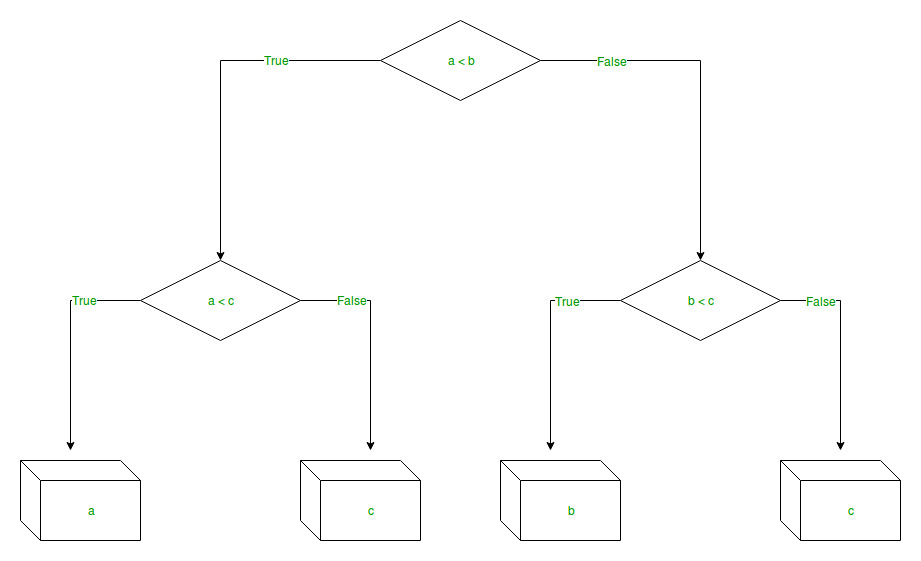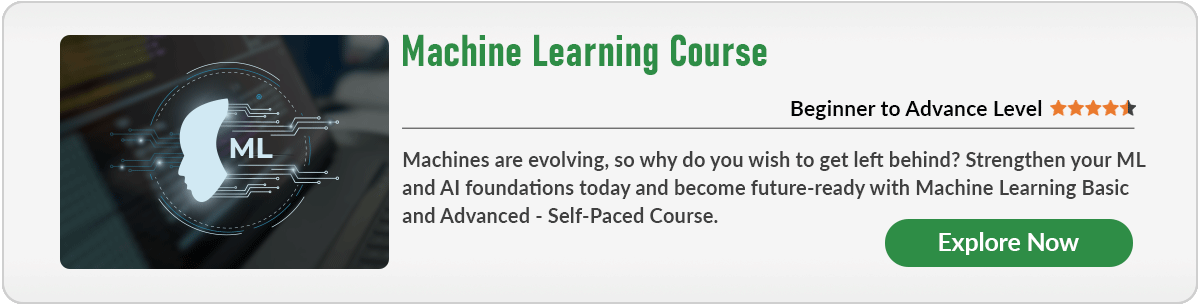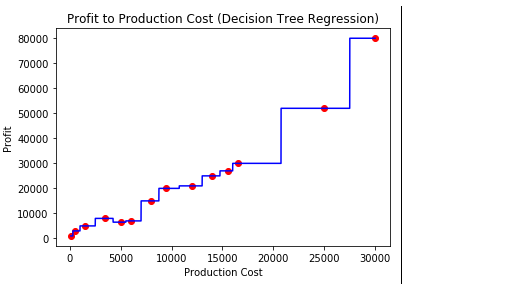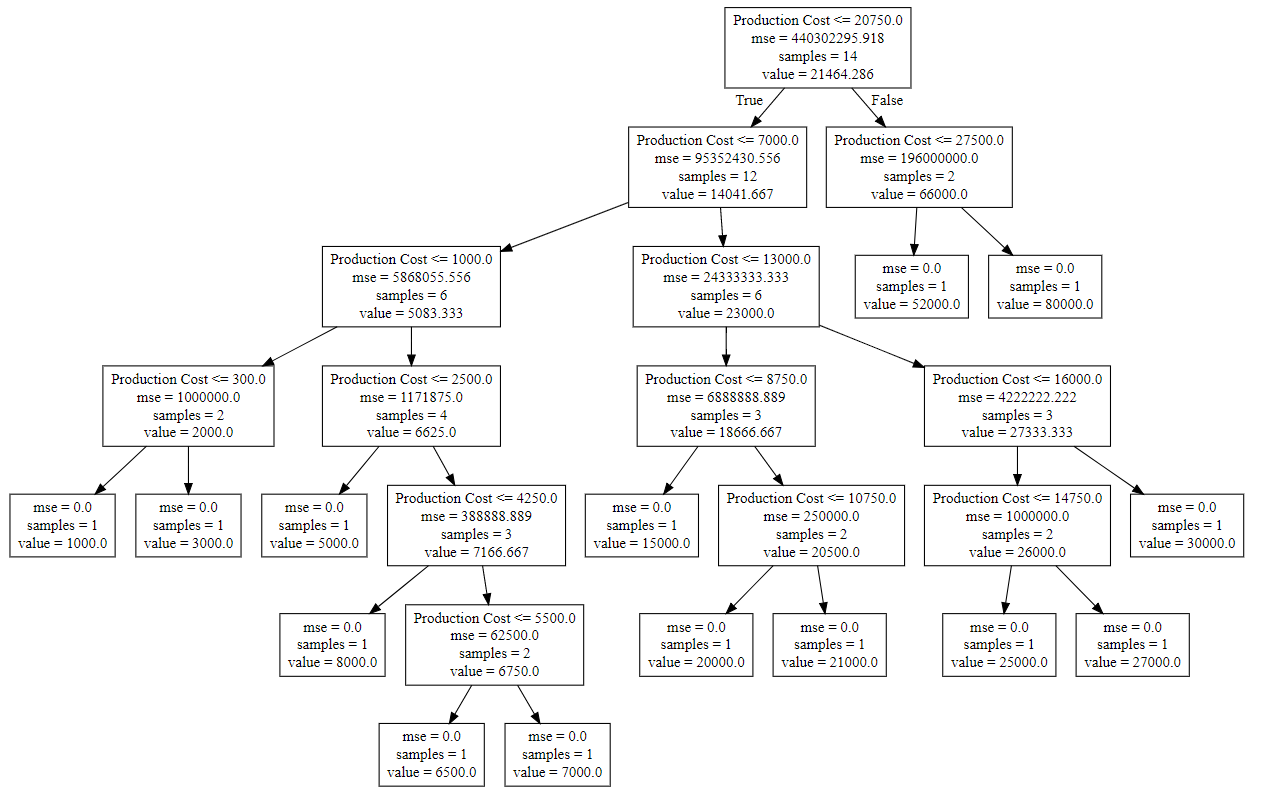# Python | Decision Tree Regression using sklearn

• Difficulty Level : Basic
• Last Updated : 23 Aug, 2022

Decision Tree is a decision-making tool that uses a flowchart-like tree structure or is a model of decisions and all of their possible results, including outcomes, input costs, and utility.
Decision-tree algorithm falls under the category of supervised learning algorithms. It works for both continuous as well as categorical output variables.

The branches/edges represent the result of the node and the nodes have either:

1. Conditions [Decision Nodes]
2. Result [End Nodes]

The branches/edges represent the truth/falsity of the statement and take makes a decision based on that in the example below which shows a decision tree that evaluates the smallest of three numbers:Decision Tree Regression:
Decision tree regression observes features of an object and trains a model in the structure of a tree to predict data in the future to produce meaningful continuous output. Continuous output means that the output/result is not discrete, i.e., it is not represented just by a discrete, known set of numbers or values.

Discrete output example: A weather prediction model that predicts whether or not there’ll be rain on a particular day.
Continuous output example: A profit prediction model that states the probable profit that can be generated from the sale of a product.
Here, continuous values are predicted with the help of a decision tree regression model.

Let’s see the Step-by-Step implementation –

• Step 1: Import the required libraries.

## Python3

 `# import numpy package for arrays and stuff ` `import` `numpy as np  ` ` `  `# import matplotlib.pyplot for plotting our result ` `import` `matplotlib.pyplot as plt ` ` `  `# import pandas for importing csv files  ` `import` `pandas as pd  `

• Step 2: Initialize and print the Dataset.

## Python3

 `# import dataset ` `# dataset = pd.read_csv('Data.csv')  ` `# alternatively open up .csv file to read data ` ` `  `dataset ``=` `np.array( ` `[[``'Asset Flip'``, ``100``, ``1000``], ` `[``'Text Based'``, ``500``, ``3000``], ` `[``'Visual Novel'``, ``1500``, ``5000``], ` `[``'2D Pixel Art'``, ``3500``, ``8000``], ` `[``'2D Vector Art'``, ``5000``, ``6500``], ` `[``'Strategy'``, ``6000``, ``7000``], ` `[``'First Person Shooter'``, ``8000``, ``15000``], ` `[``'Simulator'``, ``9500``, ``20000``], ` `[``'Racing'``, ``12000``, ``21000``], ` `[``'RPG'``, ``14000``, ``25000``], ` `[``'Sandbox'``, ``15500``, ``27000``], ` `[``'Open-World'``, ``16500``, ``30000``], ` `[``'MMOFPS'``, ``25000``, ``52000``], ` `[``'MMORPG'``, ``30000``, ``80000``] ` `]) ` ` `  `# print the dataset ` `print``(dataset)  `

Output:

```[['Asset Flip' '100' '1000']
['Text Based' '500' '3000']
['Visual Novel' '1500' '5000']
['2D Pixel Art' '3500' '8000']
['2D Vector Art' '5000' '6500']
['Strategy' '6000' '7000']
['First Person Shooter' '8000' '15000']
['Simulator' '9500' '20000']
['Racing' '12000' '21000']
['RPG' '14000' '25000']
['Sandbox' '15500' '27000']
['Open-World' '16500' '30000']
['MMOFPS' '25000' '52000']
['MMORPG' '30000' '80000']]```
• Step 3: Select all the rows and column 1 from the dataset to “X”.

## Python3

 `# select all rows by : and column 1 ` `# by 1:2 representing features ` `X ``=` `dataset[:, ``1``:``2``].astype(``int``)  ` ` `  `# print X ` `print``(X) `

Output:

```[[  100]
[  500]
[ 1500]
[ 3500]
[ 5000]
[ 6000]
[ 8000]
[ 9500]





]
```
• Step 4: Select all of the rows and column 2 from the dataset to “y”.

## Python3

 `# select all rows by : and column 2 ` `# by 2 to Y representing labels ` `y ``=` `dataset[:, ``2``].astype(``int``)  ` ` `  `# print y ` `print``(y) `Output:

`[ 1000  3000  5000  8000  6500  7000 15000 20000 21000 25000 27000 30000 52000 80000]`
• Step 5: Fit decision tree regressor to the dataset

## Python3

 `# import the regressor ` `from` `sklearn.tree ``import` `DecisionTreeRegressor  ` ` `  `# create a regressor object ` `regressor ``=` `DecisionTreeRegressor(random_state ``=` `0``)  ` ` `  `# fit the regressor with X and Y data ` `regressor.fit(X, y) `

Output:

```DecisionTreeRegressor(ccp_alpha=0.0, criterion='mse', max_depth=None,
max_features=None, max_leaf_nodes=None,
min_impurity_decrease=0.0, min_impurity_split=None,
min_samples_leaf=1, min_samples_split=2,
min_weight_fraction_leaf=0.0, presort='deprecated',
random_state=0, splitter='best')```
• Step 6: Predicting a new value

## Python3

 `# predicting a new value ` ` `  `# test the output by changing values, like 3750 ` `y_pred ``=` `regressor.predict([[``3750``]]) ` ` `  `# print the predicted price ` `print``(``"Predicted price: % d\n"``%` `y_pred)  `

Output:

`Predicted price:  8000`
• Step 7: Visualising the result

## Python3

 `# arange for creating a range of values  ` `# from min value of X to max value of X  ` `# with a difference of 0.01 between two ` `# consecutive values ` `X_grid ``=` `np.arange(``min``(X), ``max``(X), ``0.01``) ` ` `  `# reshape for reshaping the data into  ` `# a len(X_grid)*1 array, i.e. to make ` `# a column out of the X_grid values ` `X_grid ``=` `X_grid.reshape((``len``(X_grid), ``1``))  ` ` `  `# scatter plot for original data ` `plt.scatter(X, y, color ``=` `'red'``) ` ` `  `# plot predicted data ` `plt.plot(X_grid, regressor.predict(X_grid), color ``=` `'blue'``)  ` ` `  `# specify title ` `plt.title(``'Profit to Production Cost (Decision Tree Regression)'``)  ` ` `  `# specify X axis label ` `plt.xlabel(``'Production Cost'``) ` ` `  `# specify Y axis label ` `plt.ylabel(``'Profit'``) ` ` `  `# show the plot ` `plt.show() `• Step 8: The tree is finally exported and shown in the TREE STRUCTURE below, visualized using http://www.webgraphviz.com/ by copying the data from the ‘tree.dot’ file.

## Python3

 `# import export_graphviz ` `from` `sklearn.tree ``import` `export_graphviz  ` ` `  `# export the decision tree to a tree.dot file ` `# for visualizing the plot easily anywhere ` `export_graphviz(regressor, out_file ``=``'tree.dot'``, ` `               ``feature_names ``=``[``'Production Cost'``])  `

Output (Decision Tree):My Personal Notes arrow_drop_up
Recommended Articles
Page :